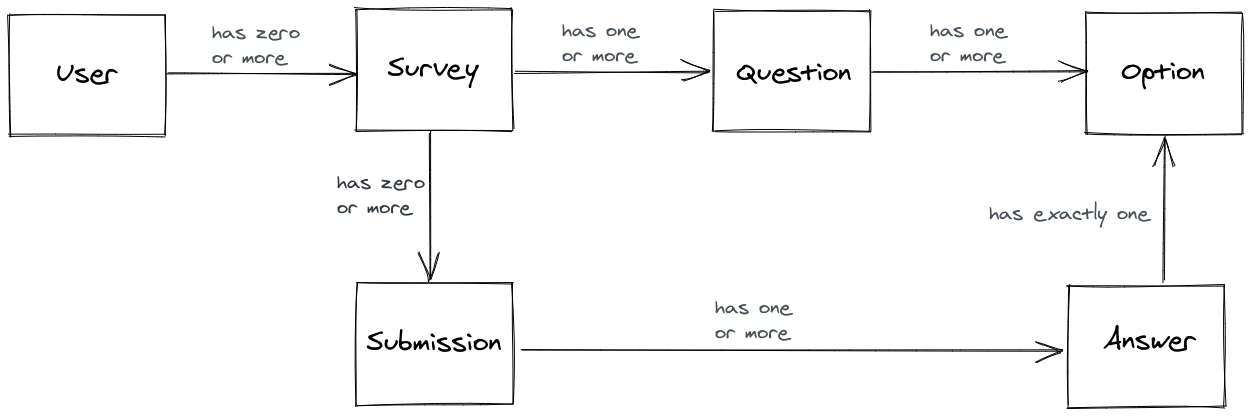# Django project blueprint: data model

Sat 03 October 2020, by Matthew Segal
Category: Appendix

This post is an appendix to my post on designing a Django project. In this page I explain why I chose to use this data model:I created this data model by looking at the user journeys and thinking about what data I would need to make them work. Here's the thought process I used. First I thought about the data that I need to define everything about a "survey" in the app. I decided that I would need:

• a Survey model to represent each survey; and then
• a link between each Survey and a User, since we need to restrict survey access to only the user who owns it
• a Question model for each question on the survey. Each survey needs to have one or more questions, so we can't hardcode questions as fields on the Survey model, so we must create a new Question model which knows which survey owns it
• each Question has one re more multi-choice answer options, so we must create an Option model

Next, I thought about how we would record a survey taker answering the questions. We would need:

• a Submission model to represent each survey taker's submission
• a link between Submission and Survey, so each submission can know which survey it belongs to
• the Answers to each question, where the answer is for a particular Option

If you have any feedback or questions email me at [email protected]